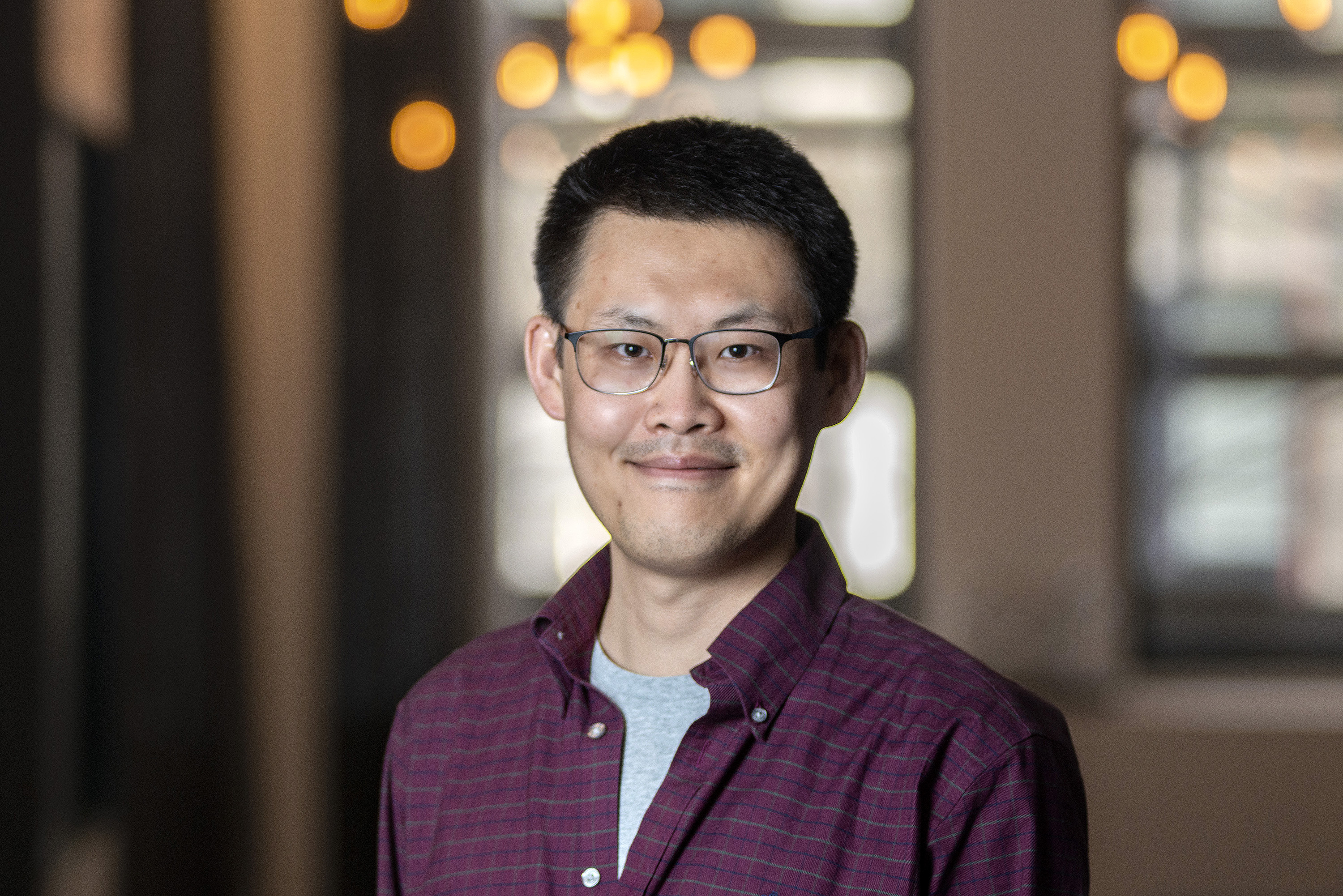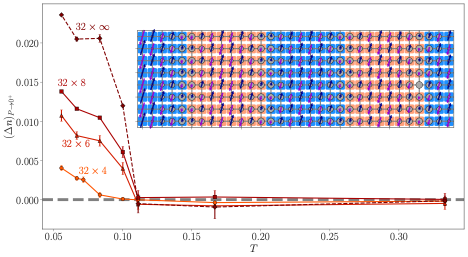# Capturing the Quantum Essence: Solving Simplified Models with High Precision

At the Flatiron Institute, computational quantum physicist Bo Xiao is working to understand the importance of materials’ fundamental properties by solving simplified mathematical models on an infinite grid.Bo Xiao is a Flatiron Research Fellow in the Center for Computational Quantum Physics Credit: John Smock

Ever since his early school years, Bo Xiao has had a knack for math and physics. A curiosity for unraveling the intricacies of the world and a love of solving intellectual puzzles made a career as a scientist an obvious choice.

Today, he still likes to be challenged by tough problems — though those problems are much more complicated than those he faced in high school. Xiao now works as a computational quantum physicist at the Flatiron Institute, tackling complex problems such as solving mathematical models that capture essential features of quantum materials. This work could help researchers understand the fundamental laws governing quantum systems — enabling leaps in technology such as predicting new materials and developing quantum computing.

Xiao joined the Center for Computational Quantum Physics (CCQ) as a Research Fellow in 2020. Before that, he earned a bachelor’s degree at Wuhan University, a master’s degree from Michigan State University, and a doctorate in physics from the University of California, Davis.

Xiao recently spoke to the Simons Foundation about his work and the importance of fundamental models. The conversation has been edited for length and clarity.

I’m working to solve mathematical models of particles interacting on lattices, which are grid-like frameworks in two or three dimensions that help describe the movement and interaction of particles. Solving lattice models, either exactly or with approximations, can help us understand the properties of real-world quantum materials, such as superconductors, which can conduct electric current without any resistance. Superconductors have many real-world applications, from medical imaging to quantum computers.

Conventional superconductors, which were first described in the 1950s using the Bardeen-Cooper-Schrieffer (BCS) theory, need to be cooled almost to absolute zero — hundreds of degrees below zero Fahrenheit — to be superconducting. The Nobel Prize-winning BCS theory describes the underlying mechanism behind the superconducting behavior observed in these materials: electrons binding into pairs through relatively weak attractions caused by vibrations of the lattice.

In 1986, researchers discovered that crystals consisting of layers of copper, oxygen and other elements can be superconducting at much higher temperatures than conventional superconductors. Some work even at minus 70 degrees Celsius. In the past four decades, researchers have attempted to find a theory that describes these superconducting materials, called cuprates. However, the mechanism underlying the high-temperature superconductivity exhibited by cuprates has yet to be definitively established.

Numerous theories and lattice models have been proposed in attempts to unravel this puzzle. Among them is the Hubbard model, which is widely regarded as the best fundamental framework describing the essential aspects of high-temperature superconductivity in cuprates. This model depicts the interaction of electrons on an infinite grid, each of whose points can hold zero, one or two electrons. Along with several colleagues at the Flatiron Institute, I am trying to solve models, including the Hubbard model, with high precision by employing advanced numerical techniques. Our goal is to illuminate new insights into high-temperature superconductivity by understanding and predicting properties of these quantum materials.

What goes into solving these models?

If we apply fundamental laws in quantum theory to describe these materials exactly, it leads to equations much too complicated to solve. Instead, I’m working with simplified lattice models. This simplification prevents us from having to account for every single detail of the quantum materials. It also allows us to solve these lattice models, gaining a profound understanding of the essential aspects of the materials with remarkable precision.

Despite the simplification, it is still a formidable challenge to achieve accurate solutions. So far, the Hubbard model can only be solved in one, infinite dimension. However, the models that we’re most interested in have two or three dimensions. In a two-dimensional Hubbard model, the complexity of solving the problem increases exponentially with the size of the lattice we study. Accurate solutions for these two-dimensional Hubbard models for the very large lattice sizes needed to understand superconductivity are still controversial after many years study.

To deal with that complexity, one class of algorithms we employ is called quantum Monte Carlo, which uses random sampling to compute an approximate answer to the problem based on probability theory. Additionally, when we want to investigate other aspects of these problems, such as real-time dynamics, we have to employ another class of algorithms called tensor networks. Tensor network algorithms are grounded in a fundamental physical concept known as entanglementwhich describes how quantum particles can become deeply connected with each other. I find this work exciting because it requires developing and coding new numerical algorithms and investigating the physical and mathematical properties of these lattice models. Being at the Flatiron Institute has been amazing because of all the resources I have at my fingertips, such as the computing resources, collaborative environment, and most importantly, the expertise of a wide range of researchers.

What are the practical applications of these models?

Solving lattice models and figuring out connections between models and experiments gives important insight into understanding electronic correlations, which can reveal unique properties of quantum materials. This can aid in the design of materials for energy-related applications, such as solar panels and batteries. This work can also help us understand the interactions between qubits, or quantum bits, which are the base unit for quantum information.

Indeed, superconductivity is not the only property we have been looking at. In our recent work, we investigate intrinsic properties of electrons, such as spin and charge, in the two-dimensional Hubbard model, and find deep connections as well as subtle differences between the model and copper-oxide materials.Depiction of the arrangement of electron charges and spins at low temperatures in the two-dimensional Hubbard model on lattices. The electrons spontaneously form striped regions with periodic modulation of spins and charges. Curves show the order parameter (a quantity that distinguishes ordered states from disordered ones) for the charge modulation as a function of temperature on difference lattice sizes. Inset shows a real-space snapshot of intrinsic properties, including spin and charge, of interacting electrons. Credit: B. Xiao, Y. He, A. Georges, and S. Zhang, Phys. Rev. X 13, 011007 (2023)

Our work is also helpful to experimentalists who work with ultracold quantum gases. Using an optical lattice — which is created by a grid of intersecting lasers — experimentalists can trap atoms in a gas. These experiments are physically very similar to the intricate quantum systems we compute using our algorithms. As a result, our numerical computations play a crucial role in benchmarking and understanding these experiments.

Where do you hope to take this work next?

As I mentioned, we need to make approximations and simplifications in order to simulate complex quantum systems, but going forward we’re working to add back in some of the things we had to remove to make the system simple. As we continue to refine our numerical algorithms and use more powerful computational resources, we can reintroduce some important ingredients originally left out, enabling us to capture a broader range of phenomena exhibited by quantum materials. For example, in the Hubbard model I mentioned earlier, we can add other important ingredients, including longer-range electron interactions and lattice vibrations known as phonons.

Additionally, I am genuinely thrilled about the notion of developing classical algorithms inspired by ideas from quantum computing. We can take ideas being developed in the quantum computing community and adapt them to our classical algorithms to help them run more efficiently on regular classical computers. I am equally fascinated by the synergistic relationship between quantum computation and classical computation through the utilization of tensor networks. My plan is to continually push forward in benchmarking quantum simulations with classical methods — enhancing classical algorithms by incorporating insights that were inspired by quantum simulations to study quantum spin models.